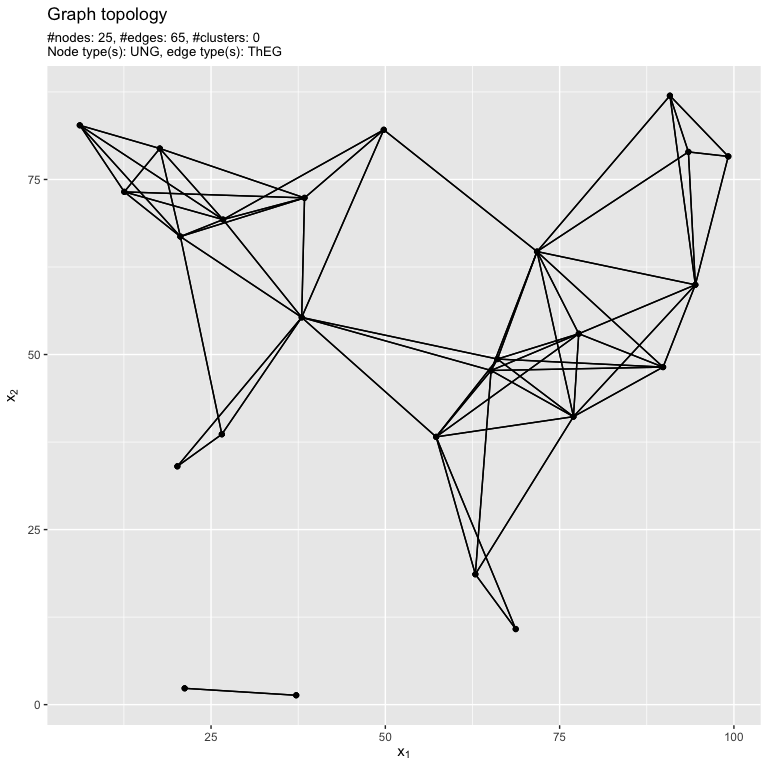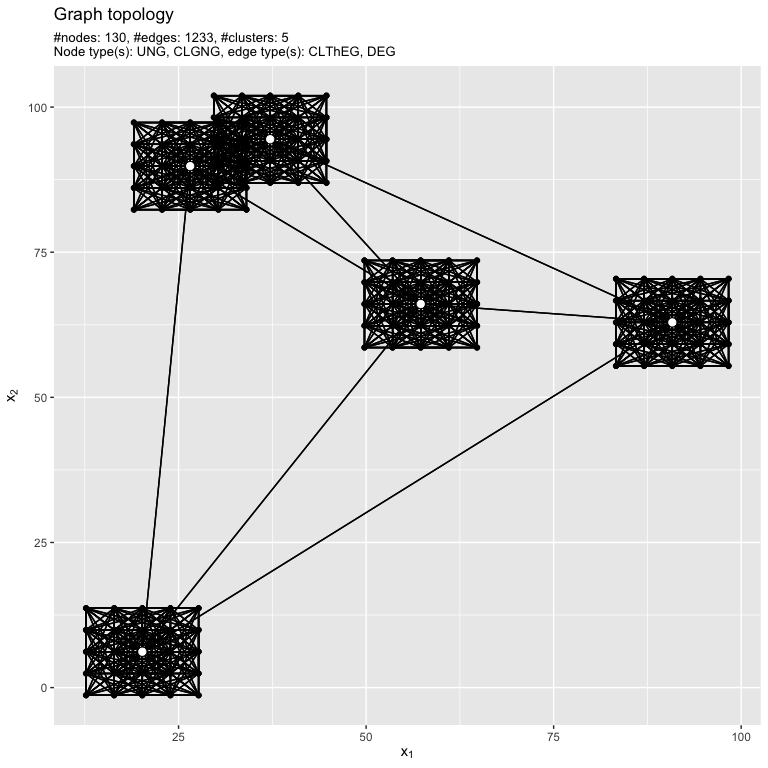# Custom generators

The graherator package already provides several node, edge and weight generators respectively. However, the collection is by far not complete and it does not aim to be. Therefore the user interface enables the definition of custom generators for each of the three steps (see basic graph generation vignette). A generator is a simple function myGenerator = function(graph, …). The first argument needs to be a grapherator object. More arguments are possible, but optional. Each generator must return a named list. The first field must be either coords (matrix of node coordinates) for node generators, adj.mat (logical matrix defining the adjacency matrix) or weights (numeric matrix of weights or list of numeric matrices) for weight generators respectively. The second field is named generator and contains a short string representation of the generator, e.g., DEG for Delauney Edge Generator.

In the following we demonstrate the definition of a custom edge generator, namely the Threshold Edge Generator. Given a graph and a positive numeric argument threshold this edge generator computes the pairwise Euclidean distances between the nodes and only keeps the edges whose distance is below the threshold value.

library(grapherator)

addEdgesThreshold = function(graph, threshold, ...) {
n = getNumberOfNodes(graph)
coords = getNodeCoordinates(graph)

# compute distances
dists = as.matrix(dist(coords, method = "euclidean", ...))

# define adjacency matrix
adj.mat = dists < threshold

# no loops allowed
diag(adj.mat) = FALSE

# return required format
return(list(adj.mat = adj.mat, generator = "ThEG"))
}

Next, we create an example graph utilizing our custom edge generator.

set.seed(1) # reproducability
g = graph(lower = 0, upper = 100)
g = addNodes(g, n = 25, generator = addNodesUniform)
g = addEdges(g, generator = addEdgesThreshold, threshold = 30)
# weight generation is skipped here
plot(g)$pl.coordsExample network. Due to smart internals the custom generators automatically work for intra- or intercluster linking without any adaptations. In the next example, we create a clustered network, apply the threshold edge generator for each cluster separately and connect cluster centers utilizing another edge generator. set.seed(1) # reproducability g = graph(lower = 0, upper = 100) g = addNodes(g, n = 5, generator = addNodesUniform) g = addNodes(g, n = 25, by.centers = TRUE, generator = addNodesGrid, lower = c(0, 0), upper = c(15, 15)) g = addEdges(g, generator = addEdgesThreshold, threshold = 13, type = "intracluster") g = addEdges(g, generator = addEdgesDelauney, type = "intercenter") # weight generation is skipped here plot(g)$pl.coordsExample network.